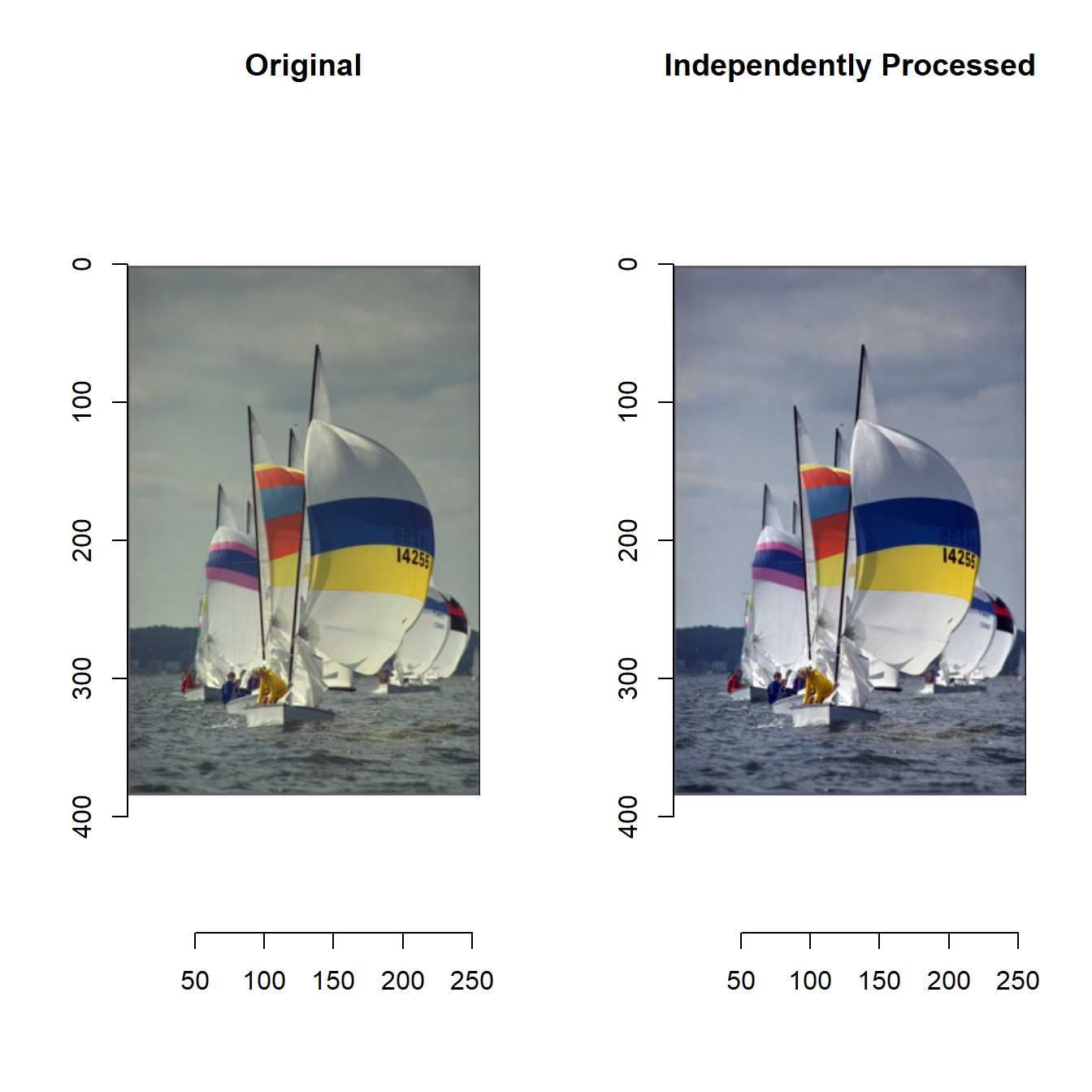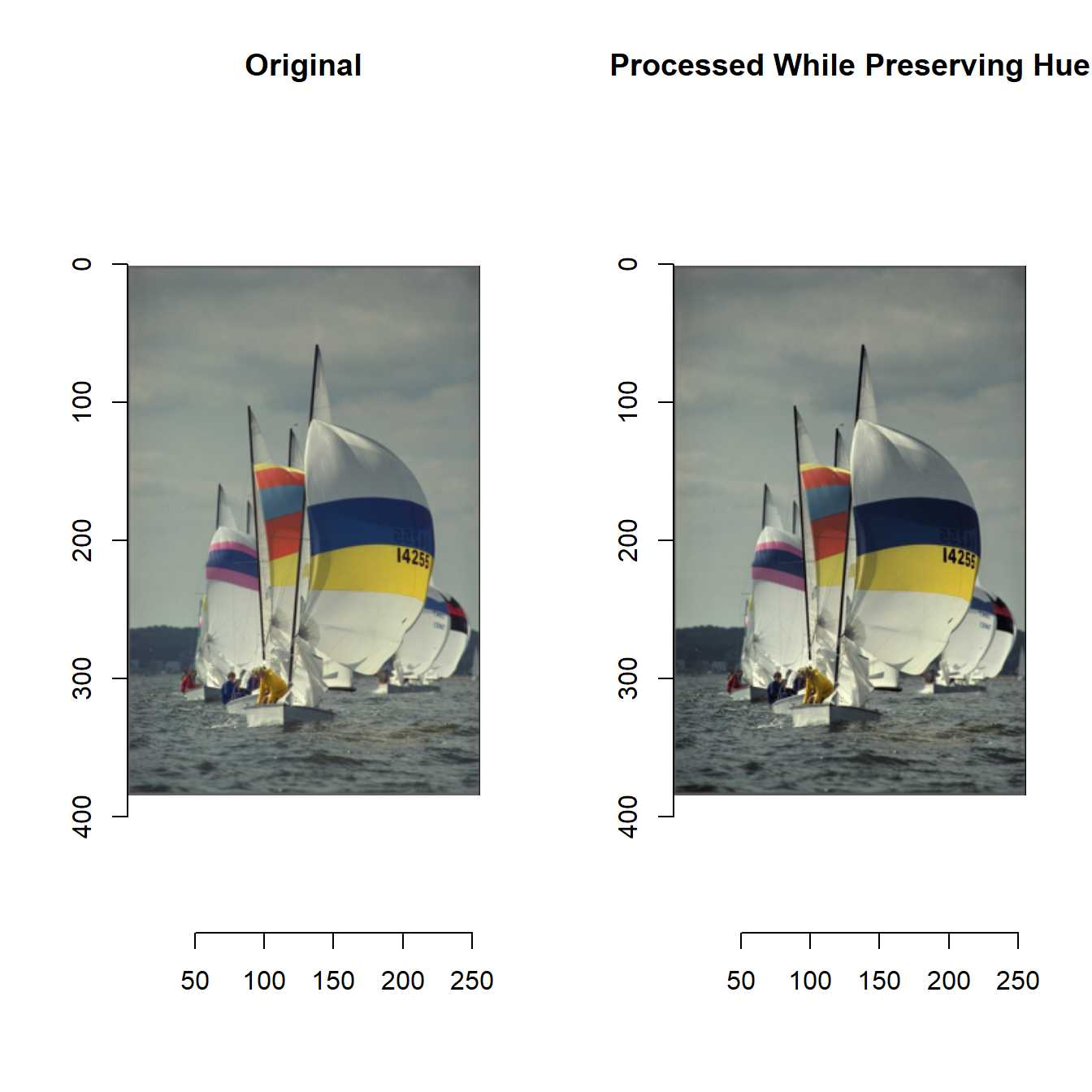Treating color image with imagerExtra

The functions of imagerExtra are basically for grayscale image.

Then can’t we treat color images with imagerExtra?

Yes, we can!

We have two options.

• process the channels independently

• preserve the hue of image, process the intensity component and then compute RGB values from the new intensity component

The former is straightforward.

One example is shown below.

library(imagerExtra)
x <- boats
s <- 0.1
R(x) <- BalanceSimplest(R(x), s, s, range=c(0,1))
G(x) <- BalanceSimplest(G(x), s, s, range=c(0,1))
B(x) <- BalanceSimplest(B(x), s, s, range=c(0,1))
layout(matrix(1:2, 1, 2))
plot(boats, main = "Original")
plot(x, main = "Independently Processed")The latter needs three functions: Grayscale, GetHue, RestoreHue.

Grayscale computes average of RGB channel.

GetHue stores hue of image.

RestoreHue restores hue of image.

Grayscale <- function(im) {
sumRGB <- (R(im) + G(im) + B(im)) / 3
}

GetHue <- function(im) {
sumRGB <- R(im) + G(im) + B(im)
pixels0 <- where(sumRGB == 0)
at(sumRGB, pixels0[,"x"], pixels0[,"y"]) <- 1
R(res) <- R(im) / sumRGB
G(res) <- G(im) / sumRGB
B(res) <- B(im) / sumRGB
return(res)
}

RestoreHue <- function(gim, hueim) {
R(res) <- gim * R(hueim)
G(res) <- gim * G(hueim)
B(res) <- gim * B(hueim)
return(res)
}

Note that grayscale of imager computes as below by default.

Y = 0.300000R + 0.590000G + 0.110000B

This equation reflects the way of human visual perception.

This grayscale conversion makes it difficult to restore hue of image.

That’s why we need Grayscale, which just compute average of RGB channels.

How to use these functions is shown below.

g <- Grayscale(boats)
hueim <- GetHue(boats)
g <- BalanceSimplest(g, s, s, range=c(0,1))
y <- RestoreHue(g, hueim)
layout(matrix(1:2, 1, 2))
plot(boats, main = "Original")
plot(y, main = "Processed While Preserving Hue")Which way is better?

It’s up to you.

You should consider which way is better when treating color image.

Grayscale, GetHue, and RestoreHue will be added to next version of imagerExtra.